### 0. 背景

Junbo Zhao等人提出的“基于能量的GAN”网络，其将判别器视为一个能量函数而不需要明显的概率解释，该函数可以是一个可训练的损失函数。能量函数是将靠近真实数据流形的区域视为低能量区域，而远离的视为高能量区域。和“概率GAN”相似，训练中，生成器会尽可能生成最小能量时候的伪造样本，而此时判别器会被赋值为高能量（因为是伪造的）。通过将判别器看成一个能量函数，就可以使用更多更广泛的网络结构和损失函数，而不只是logistic输出的二值分类器。其中Junbo Zhao等人基于此原理，提出了其中一个实现案例，即自动编码器结构，能量就是重构时候的误差，以此来代替分类器。而且此时训练相对常规的GAN也更稳定。并提出了一个单尺度结构，其可以被训练用来生成高分辨率图片。

GAN可以以两种方式去解释：

• 主要的部分是生成器：判别器扮演着可训练的目标函数的角色。我们假设数据位于一个流形上，当生成器生成的样本被识别为处在这个流形上，它就会得到一个梯度用来指示它如何修改它的输出从而更接近这个流形。在这样的场景下，判别器会当生成的样本处在流形之外的时候对生成器进行惩罚。这可以理解成一种用于训练生成器的方法，就为了让其生成合理的输出；
• 主要的部分是判别器：生成器被训练用来生成对比样本。通过迭代和逐步的输入对比样本，生成器增强了判别器的半监督学习性能。

### 1. EBGAN

#### 1.1 一个基于能量的GAN网络结构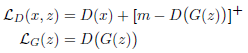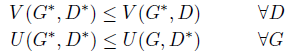• 如果$(G^*,D^*)$是系统的纳什平衡，那么在几乎任何地方都有$p_G=p_{data}$，并且此时$V(D^*,G^*)=m$(简单来说就是$D(x)=D(G(z))=0.5$)
• 该系统存在一个纳什平衡，且有：
• 几乎任何地方有$p_{G^*}=p_{data}$
• 存在一个常量$\gamma\in[0,m]$，例如$D_*(x)=\gamma$（这是假设不存在$p_{data}(x)=0$的区域，如果真的存在这种区域，那么$D^*(x)会有介于[0,m]的其他值赋予该区域$
证明在论文的2.2部分和附录A，E

#### 1.2 基于1.1的一个实现(添加正则的AE作为判别器)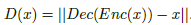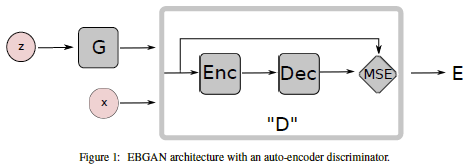• 不同于一个bit的二分类结果，基于重构的输出可以为判别器提供不同的目标。因为在二分类的logistic loss中，只有2个目标的选项，所以在一个minibatch中，对应不同样本的梯度基本上都不可能互相正交。这使得训练十分低效。另一方面，重构loss可以在一个minibatch中产生许多不同方向的梯度，从而允许更大的minibatch size基础上，基本没有loss效率的损失。
• 对于传统角度上看，ae也是用来作为基于能量的模型，所以用它也是自然选择，而且当加上正则项时，ae可以在没有监督或者负类样本的基础上学习一个能量流形。这意味着即使当一个EBGAN是基于AE实现时，可以只用真实样本来进行训练，判别器可以自己去找到数据流形。而这对于二分类的logistic loss时不可能的。

• 正则化器（生成器）可以完全训练所得，而不需要手工设计；
• 对抗训练的策略可以让生成对比样本的过程和学习能量函数的过程直接相互接触。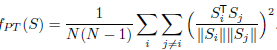PT是在一个minibatch上操作的，意在正交逐对样本表征。采用cos相似度而不是欧式距离是为了保证此项的下限值和尺度不变性。

### 2. 实验分析

#### 2.1 基于mnist的参数配置搜索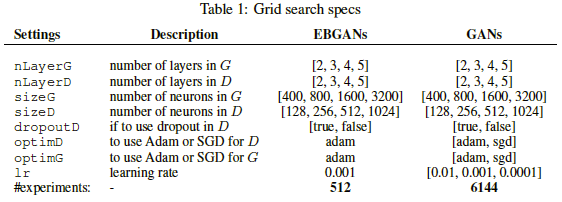• m值设定为10，且训练中无变化:
• 在每一层权重层后都有BN层，除了生曾去的输出层和判别器的输入层；
• 训练图像都缩放到[-1,1]，用来适应生成器输出层使用的tanh激活函数；
• 使用ReLU作为非线性激活函数；
• 初始化：判别器中初始化是N(0,0.002)；而生成器的是N(0,0.02)。偏置都初始化为0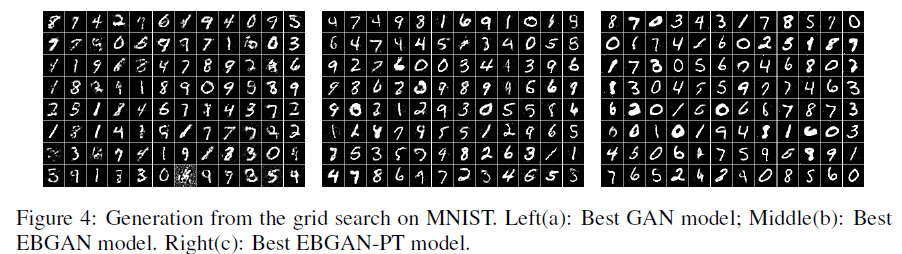• (a): $nLayerG=5, nLayerD=2, sizeG=1600, sizeD=1024, dropoutD=0,optimD=SGD, optimG=SGD, lr=0.01.$
• (b): $nLayerG=5, nLayerD=2, sizeG=800, sizeD=1024, dropoutD=0,optimD=ADAM, optimG=ADAM, lr=0.001, margin=10.$
• (c): 和（b）一样，且$\lambda_{PT} = 0.1$

#### 2.2 基于mnist的半监督学习

posted @ 2018-03-01 23:29  仙守  阅读(1555)  评论(0编辑  收藏  举报